# em 7 Draw the condensed structural formulas for the products from each of the following reactions:...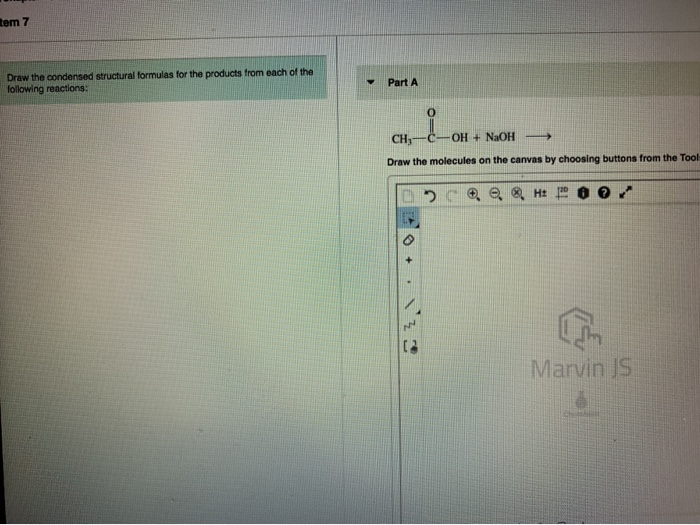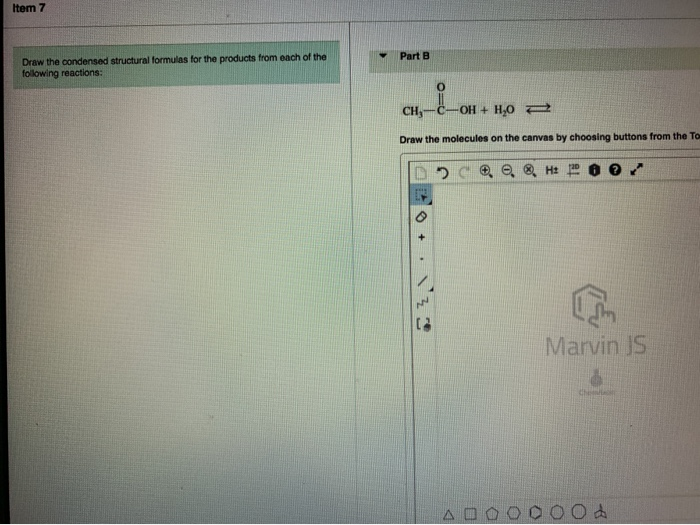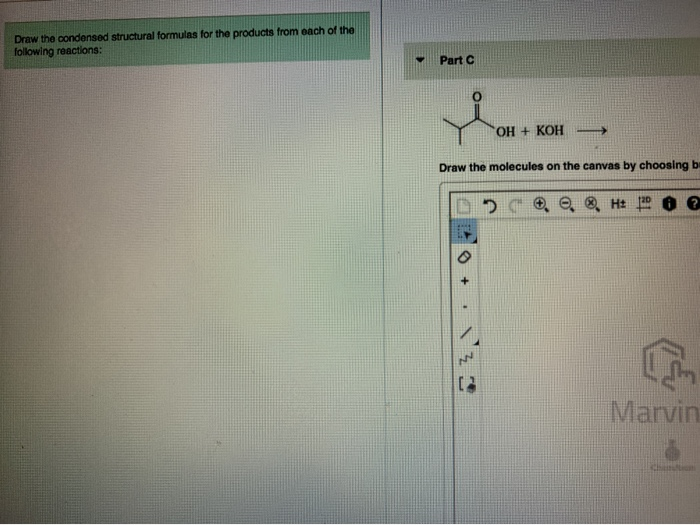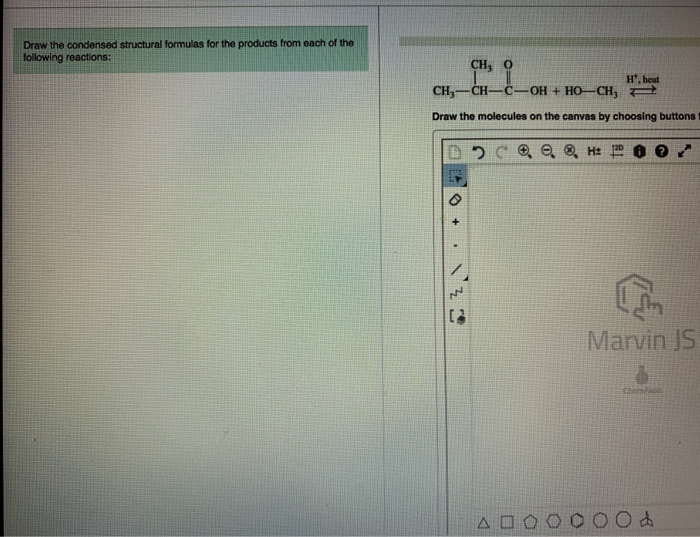em 7 Draw the condensed structural formulas for the products from each of the following reactions: Part A CH --OH + NaOH Draw the molecules on the canvas by choosing buttons from the Tool O e Hoor Marvin JS
Item 7 Part B Draw the condensed structural formulas for the products from each of the following reactions: CH3-C-OH + H2O + Draw the molecules on the canvas by choosing buttons from the To D HE POO Marvin JS sooooood
Draw the condensed structural formulas for the products from each of the following reactions: Part C OH + KOH-> Draw the molecules on the canvas by choosing b D? . H: 70 @ Marvin
Draw the condensed structural formulas for the products from each of the following reactions: CH, 0. H. heat CH-CH=C=OH + HO–CH, 4 Draw the molecules on the canvas by choosing buttons DC @ @ @ H: 80 O + VZ Marvin JS ooooood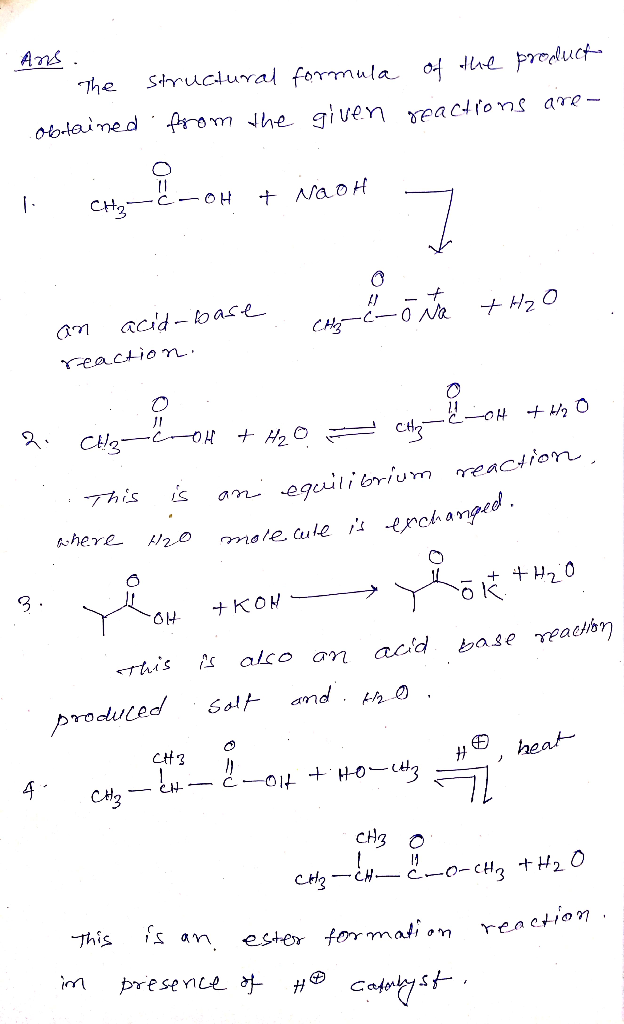#### Earn Coin

Coins can be redeemed for fabulous gifts.

Similar Homework Help Questions
• ### ▼ Part A Convert the following line-angle structural formulas to a condensed structural formula Draw the...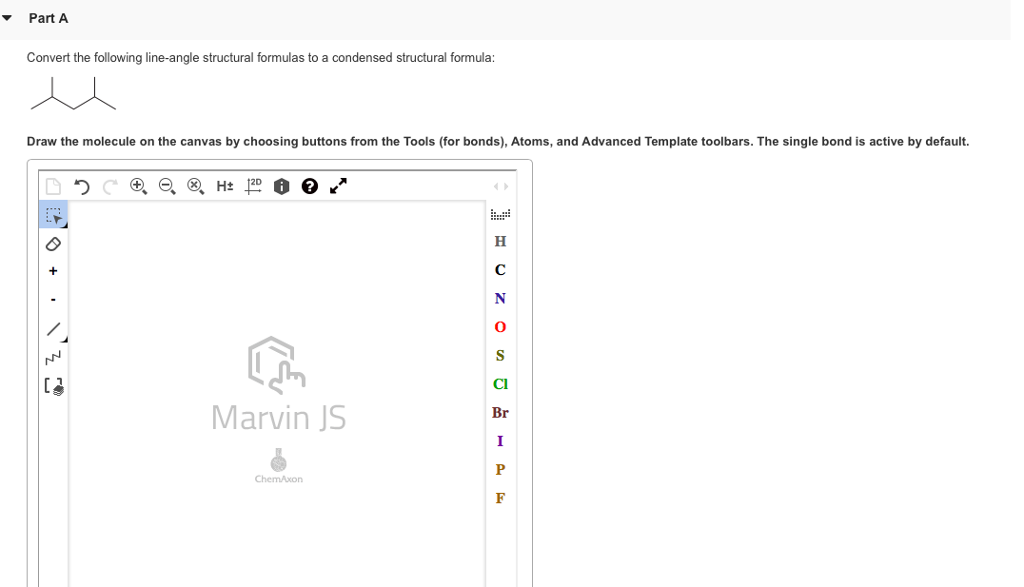▼ Part A Convert the following line-angle structural formulas to a condensed structural formula Draw the molecule on the canvas by choosing buttons from the Tools (for bonds), Atoms, and Advanced Template toolbars. The single bond is active by default 2D CI Marvin JS Br

• ### * Review Constar Draw the condensed structural formula of the ester formed when each of the...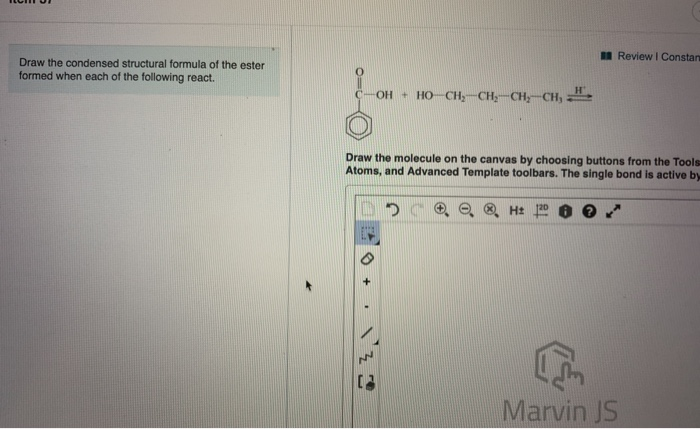* Review Constar Draw the condensed structural formula of the ester formed when each of the following react. C-OH + HOẠCH, CHỊ-CH, CH, H, Draw the molecule on the canvas by choosing buttons from the Tools Atoms, and Advanced Template toolbars. The single bond is active by ? . . H: 1200 + . Z Marvin JS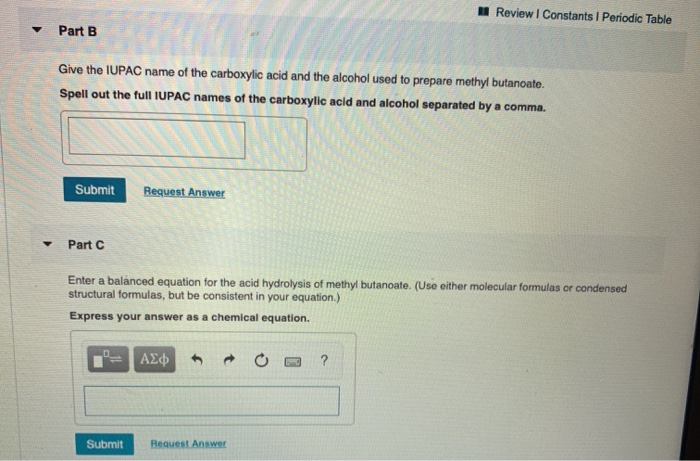please help ! answer all quetions for the 8 photos thank you! Review Constants Periodic Table Part B Give the IUPAC name of the carboxylic acid and the alcohol used to prepare methyl butanoate. Spell out the full IUPAC names of the carboxylic acid and alcohol separated by a comma. Submit Request Answer Part C Enter a balanced equation for the acid hydrolysis of methyl butanoate. (Use either molecular formulas or condensed structural formulas, but be consistent in your equation.)...

• ### Problem 14.64 5 of 20 > M Review Constants Periodic Table Draw the condensed structural or...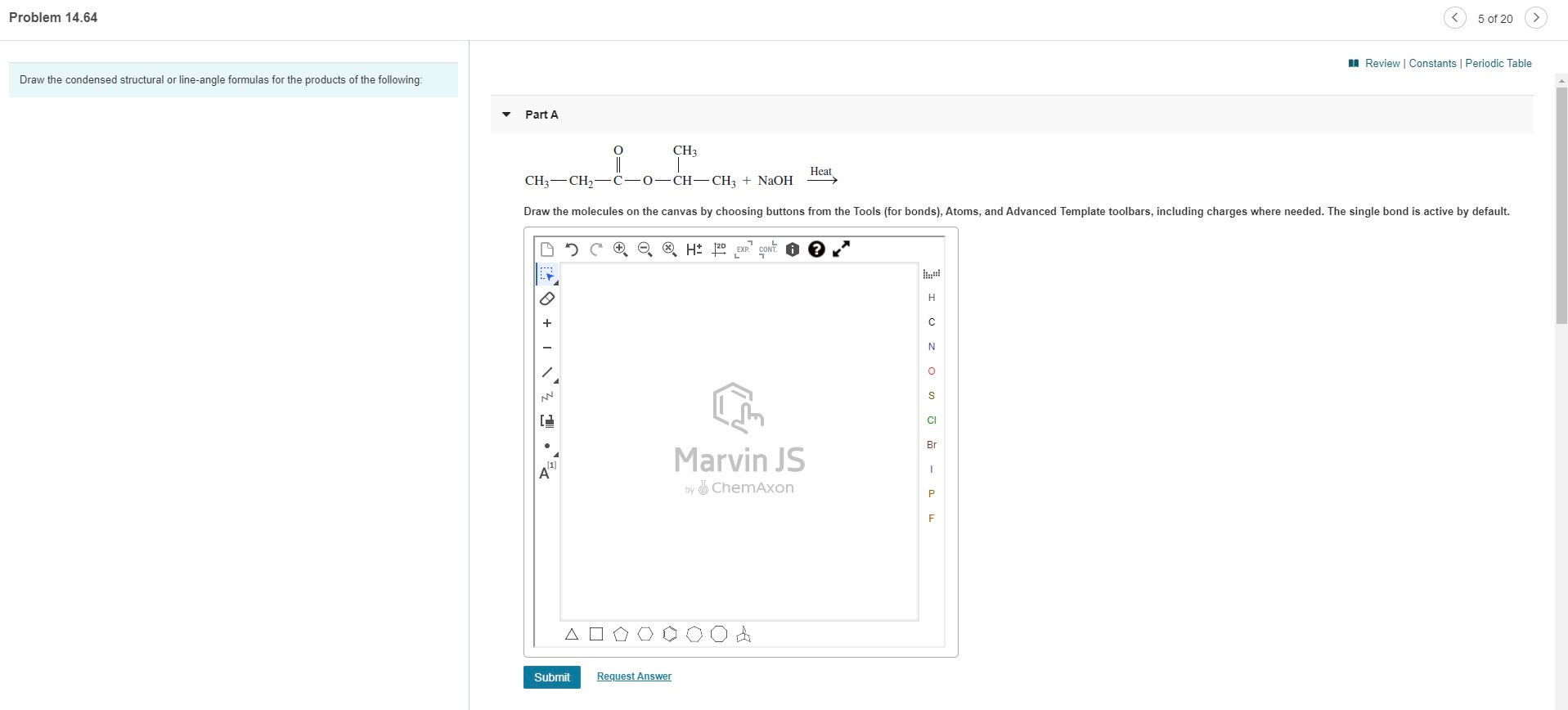Problem 14.64 5 of 20 > M Review Constants Periodic Table Draw the condensed structural or line-angle formulas for the products of the following Part A 0 CH3 Heat CH3-CH2-C-0-CH-CH3 + NaOH Draw the molecules on the canvas by choosing buttons from the Tools (for bonds), Atoms, and Advanced Template toolbars, including charges where needed. The single bond is active by default. H* 2D BXP H O + N O s CI Br Marvin JS by a chemAxon 1 A...

• ### Problem 14.64 5 of 20 A Review Constants Periodic Table Draw the condensed structural or line-angle...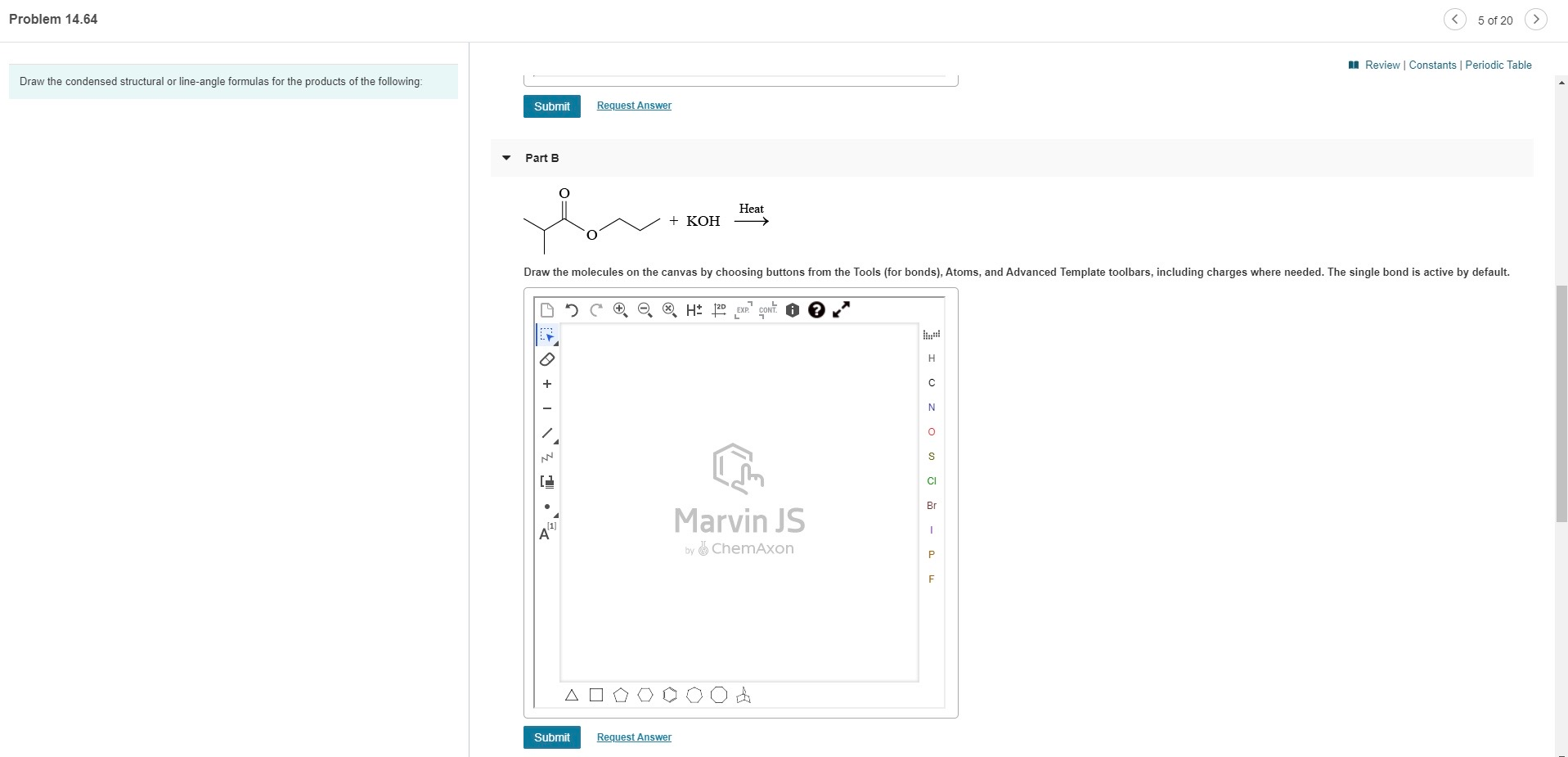Problem 14.64 5 of 20 A Review Constants Periodic Table Draw the condensed structural or line-angle formulas for the products of the following: Submit Request Answer Part B 0 Heat + KOH Draw the molecules on the canvas by choosing buttons from the Tools (for bonds), Atoms, and Advanced Template toolbars, including charges where needed. The single bond is active by default. LH2D EXP CONT. O . H с N 0 S CI Br Marvin JS (1) A 1 by...

• ### Problem 10.40 Draw the major products obtained from heating each of the following ethers with one...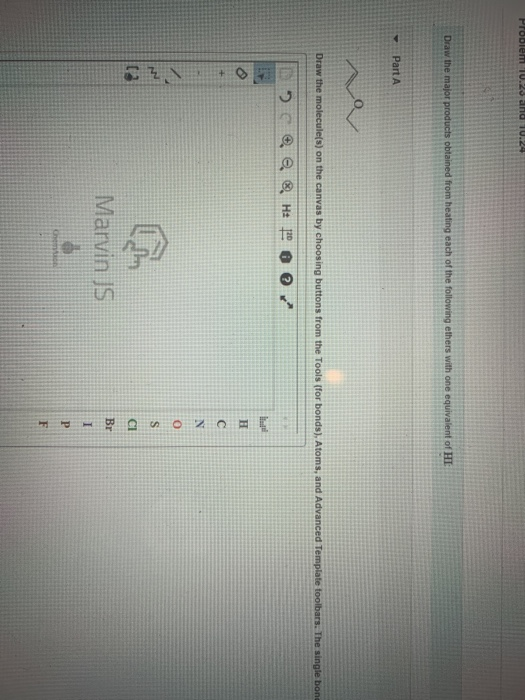Problem 10.40 Draw the major products obtained from heating each of the following ethers with one equivalent of HE Part A Draw the molecule(s) on the canvas by choosing buttons from the Tools (for bonds), Atoms, and Advanced Template toolbars. The single bona B. H: 20 @ @ ZOJA Marvin JS Part B. Draw the molecule(s) on the canvas by choosing buttons from the Tools (for bonds), Atoms, and Advanced > e® H1200 CZ,\+ 1 = 0 Zowo - A...

• ### Problem 14.64 < 5 of 20 M Review Constants Periodic Table Draw the condensed structural or...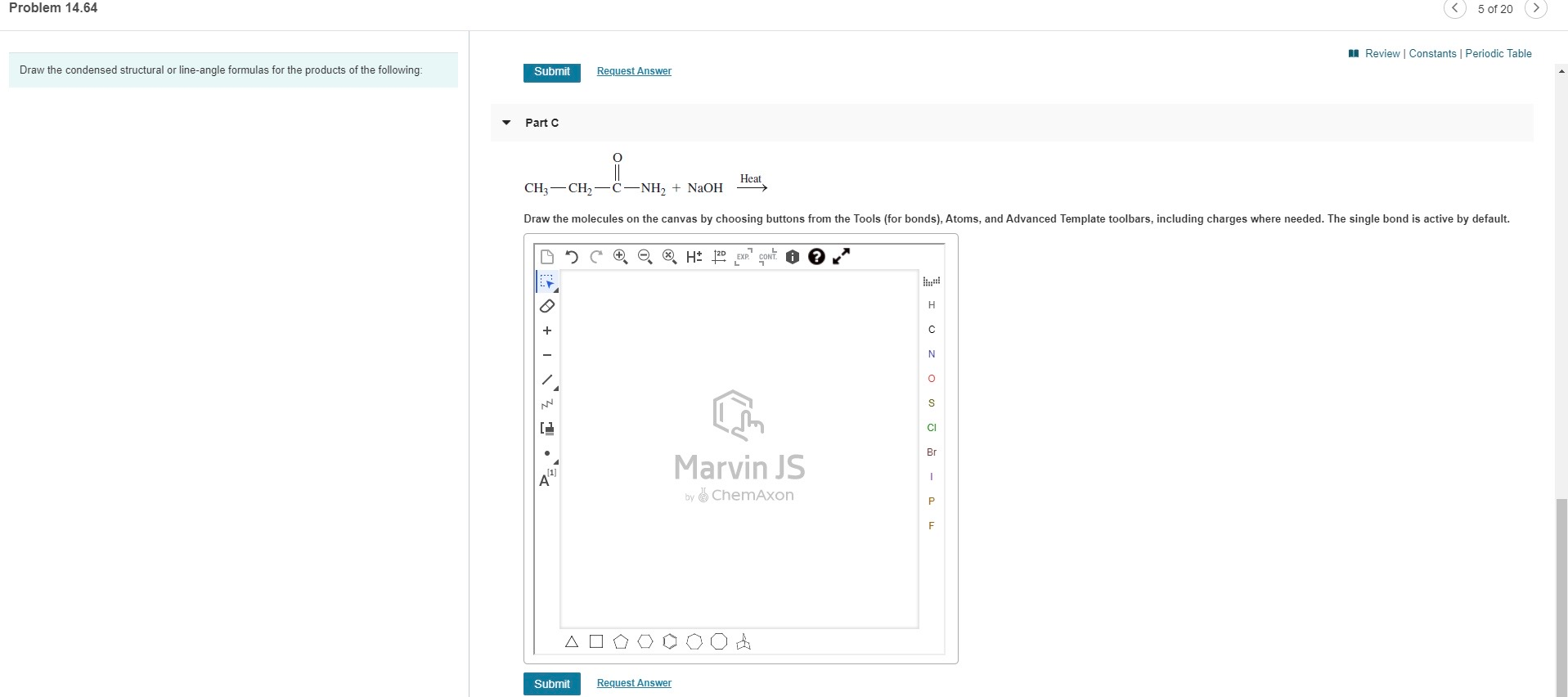Problem 14.64 < 5 of 20 M Review Constants Periodic Table Draw the condensed structural or line-angle formulas for the products of the following: Submit Request Answer Part C O=0 Heat CH3-CH2–C-NH2 + NaOH Draw the molecules on the canvas by choosing buttons from the Tools (for bonds), Atoms, and Advanced Template toolbars, including charges where needed. The single bond is active by default. H* 120 EXP" CONT H с N O S СІ Br 4 1632 Marvin JS by...

• ### Draw the condensed structural formula, or line-angle formula, if cyclic, for each of the following. Review...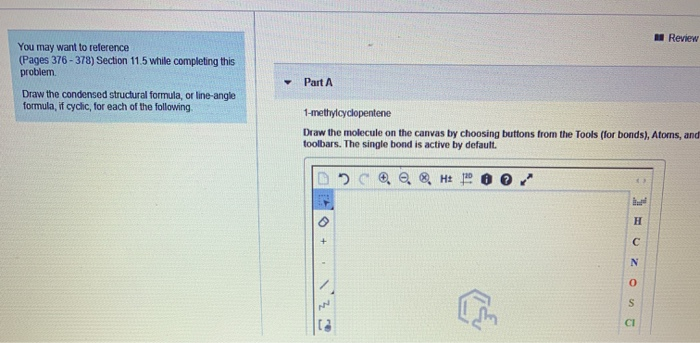Draw the condensed structural formula, or line-angle formula, if cyclic, for each of the following. Review You may want to reference (Pages 376 - 378) Section 11.5 while completing this problem Part A Draw the condensed structural formula, or line-angle formula, if cyclic, for each of the following 1-methylcyclopentene Draw the molecule on the canvas by choosing buttons from the Tools (for bonds), Ators, and toolbars. The single bond is active by default. DOCH 10 You may want to reference...

• ### nework9 olem 18.53 Draw the line-angle formula for the products obtained from the hydrolysis of the...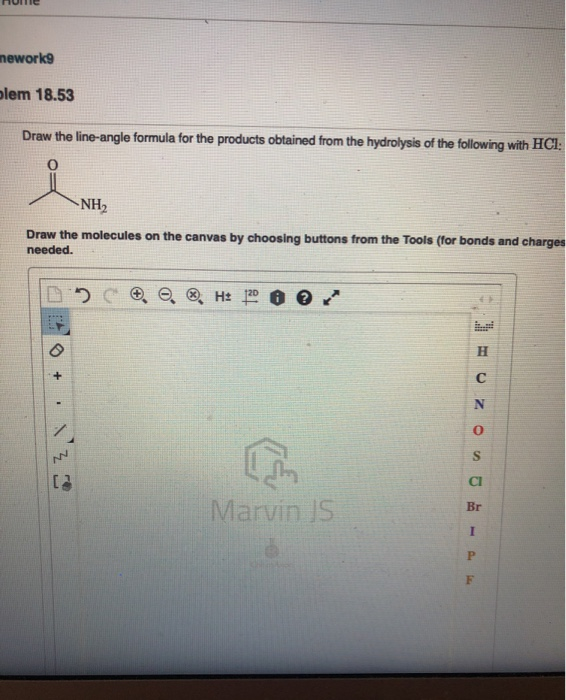nework9 olem 18.53 Draw the line-angle formula for the products obtained from the hydrolysis of the following with HCl NH2 Draw the molecules on the canvas by choosing buttons from the Tools (for bonds and charges needed. e Ht 20 + Marvin IS I Problem 18.53 Draw the condensed structural formula for the products obtained from the hydrolysis of the following with CH3-CH2-C- NH2 Draw the molecules on the canvas by choosing buttons from the Tools (for bonds and charges),...

• ### Provide the products for the following reactions: Part A o NHBr + NaOH Draw the molecules...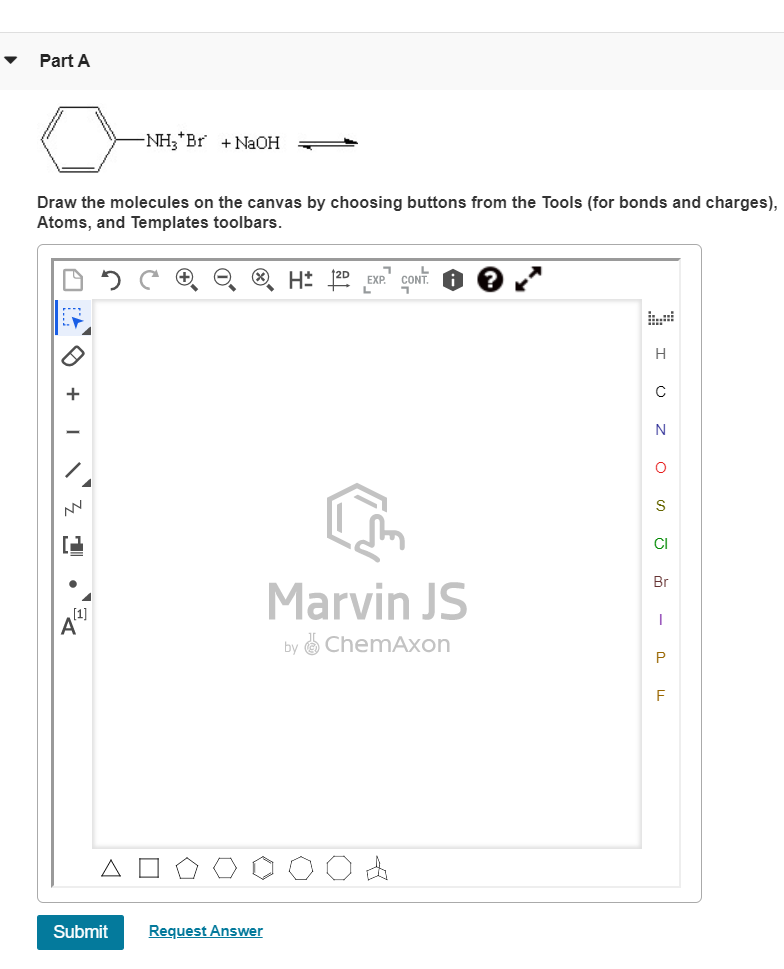Provide the products for the following reactions: Part A o NHBr + NaOH Draw the molecules on the canvas by choosing buttons from the Tools (for bonds and charges), Atoms, and Templates toolbars. H+ 120 EXP." CONT. i H с N O s CI DE Z Br Marvin JS by ChemAxon 1 Р F A Submit Request Answer Part B CH3CH2CH2 -NH3 + KOH Draw the molecules on the canvas by choosing buttons from the Tools (for bonds and charges),...

Free Homework App